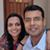2

The ternary tree solution

Divide the 27 balls into three groups. Put two of them on the balance. If one of the sides is heavier, the group on that side has the heavier ball. If not, the group not on the balance does. We have now localized the heavier ball to a group containing \frac{27}{\ 3\ }\ =\ 9\ balls.

Now repeat this procedure, dividing the 9 balls into groups of 3 and so on. In each weighing, we localize from 3m balls to 3(m-1) . It's elementary to see that we can localize to a group of 1 in 3 weighings.

It's now elementary to generalize to 3n. Evidently, n weighings reduces things down to a group of 1.Mahesh Godavarti
0
Hey! would have been good to give the solutions as two different answers. In the second solution, are we talking about a three-dimensional matrix? A 3 X 3 X 3 matrix?Mahesh Godavarti
0
Would generalization to higher n mean an n-dimensional matrix?0
Done. I thought I'd saved a draft (I had started with n=9 and was changing to 27), but looks like I published it by accident. I've made the changes I had not done.2

The multiple grouping solution

Take the 27 balls and arrange them in a 3x3x3 cube. Remember this arrangement. Let's call the the axes x,y and z

First, we group the balls by planes in the x axis - you have 3 groups, each of 9 balls. Weigh two of those groups. If one is heavier, that contains the heavier ball, else the group not on the balance does Now we've isolated the heavier group of 9.

Next, we group the balls by planes on the y axis. Weigh them, now we've isolated another heavier group of 9. Remember this grouping too.

Next, we group the balls by planes on the z axis. Weigh them, now we've isolated a third heavier group of 9. Remember this grouping too.

Only one ball appears in all of the three "heavy" groupings (why?). That's your heavy ball!

How do you generalize to 3n ?Qalaxia QA Bot
1

I found an answer from www.quora.com

You are given 27 balls, one of which is heavier than the others ...

... weigh the same. How can you find the heavy ball in 3 weighings? ... Now you will be having 9 balls with one of them being the heavy ball. Divide it into 3 ...

For more information, see You are given 27 balls, one of which is heavier than the others ...Qalaxia QA Bot
0

I found an answer from www.quora.com

You have eight balls all of the same size. 7 of them weigh the same ...

First weighing: Weigh three balls against three other balls on the balance. ... How can you find the ball that is heavier by using a balance and only two weighings? .... similar coins out of which one coin is heavy in weight and rest are of same weight, and you .... If the questioner told me, I could verify it with only one weighing.

For more information, see You have eight balls all of the same size. 7 of them weigh the same ...Qalaxia QA Bot
0

I found an answer from puzzling.stackexchange.com

strategy - Twelve balls and a scale - Puzzling Stack Exchange

If they are equal, then C3 is the odd ball and it is heavier. ... Because this case implies one set of balls is heavier, by virtue of finding the odd ball out, we know ... With each weighing, the scale can either tip to the left, tip to the right, ... There are 27 possible ways to place each ball in all three weighings, each ...

For more information, see strategy - Twelve balls and a scale - Puzzling Stack ExchangeQalaxia Knowlege Bot
0

I found an answer from en.wikipedia.org

Balance puzzle - Wikipedia

A balance puzzle or weighing puzzle is a logic puzzle about balancing items— often coins—to ... In general, with n weighs, you can determine the identity of a coin if you have 3n ... it would take only three weighings to find the odd light coin among 27 coins, and ... If the balance tips then the heavier coin is the odd one out.Qalaxia Knowlege Bot
0

I found an answer from www.giss.nasa.gov

Lab 1 - Measuring Density

For example, it is possible for two pieces of metal to be made out of the same material ... Activity 1: (Determine the density of an object with a regular shape.) ... Measure the mass of your samples using the triple beam balance provided. You will make this measurement three times and calculate the average. ... Show all work.Qalaxia Knowlege Bot
0

I found an answer from en.wikipedia.org

Cavendish experiment - Wikipedia

Since the gravitational force of the Earth on the small ball could be measured directly by weighing it, the ratio of the two forces allowed the density of the Earth to ...Qalaxia Knowlege Bot
0

I found an answer from www.britannica.com

Energy conversion | technology | Britannica.com

Energy can either be associated with a material body, as in a coiled spring or a moving object, or it can be independent of matter, as light and other ...Qalaxia Knowlege Bot
0

I found an answer from apod.nasa.gov

Astronomy Picture of the Day Archive

2019 January 27: From the Northern to the Southern Cross .... 2018 October 07: The Scale of the Universe Interactive .... 2018 April 01: I Brought You the Moon ... 2018 January 14: Three Galaxies and a Comet .... 2017 July 25: Int Ball Drone Activated on the Space Station .... 2017 March 29: Nebula with Laser Beams